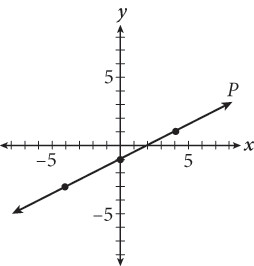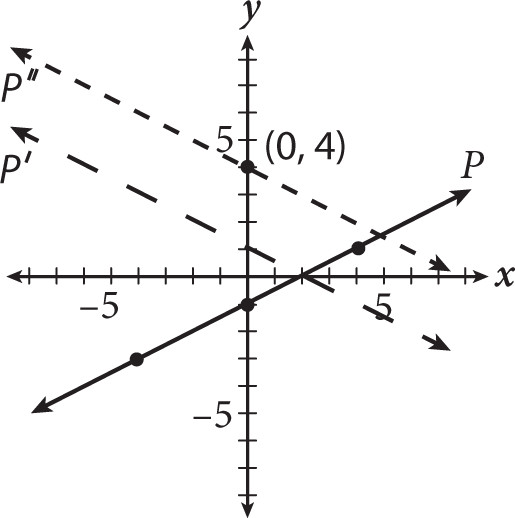# SAT Math Multiple Choice Question 337: Answer and Explanation

### Test Information

Question: 337

7.If line P shown in the graph is reflected over the x-axis and shifted up 3 units, what is the new y-intercept?

• A. (0, –4)
• B. (0, –2)
• C. (0, 2)
• D. (0, 4)

Explanation:

D

Difficulty: Medium

Category: Heart of Algebra / Linear Equations

Strategic Advice: You can approach this question conceptually or concretely. When dealing with simple transformations, drawing a quick sketch is most likely the safest approach.

Getting to the Answer: You are only concerned about the y-intercept, so keep your focus there. When the graph is reflected over the x-axis, the y-intercept will go from (0, -1) to (0, 1). Next, the line is shifted up 3 units, which adds 3 to the y-coordinates of all the points on the line, making the new y-intercept (0, 4). A sketch is provided here: